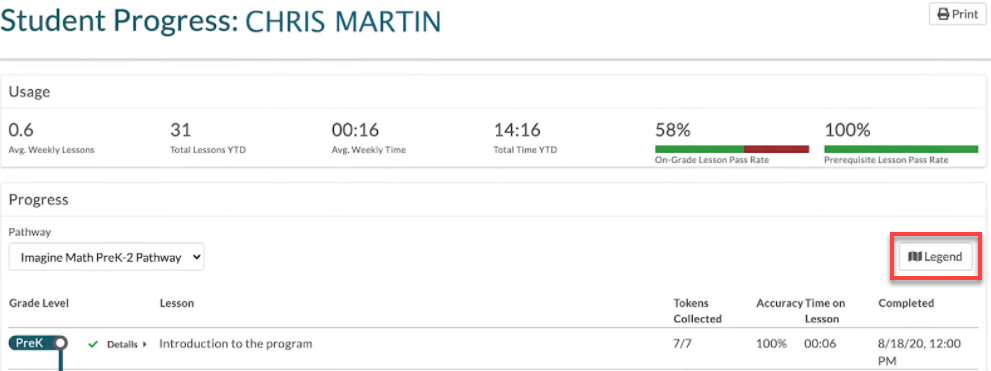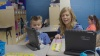# Viewing the Student Progress report in Imagine Math PreK-2

The Imagine Math PreK-2 Student Progress report provides usage and progress information for each student. You can begin using the Student Progress report as soon as your students start using Imagine Math PreK-2. Administrators and Teachers can view the Student Progress report for all students that are assigned to them. The Student Progress report updates hourly.

To view the Student Progress report:

2. In the Reports section of the navigation pane, click Student Progress.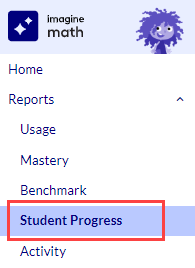3. Use the Search box or scroll through the list to find the student whose report you wish to view; then click the student's name to open the report.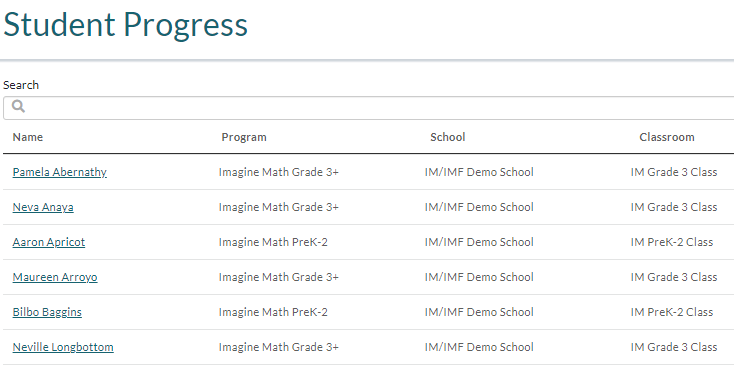## Interpreting the Student Progress Report

The Student Progress report includes a Usage and a Progress section.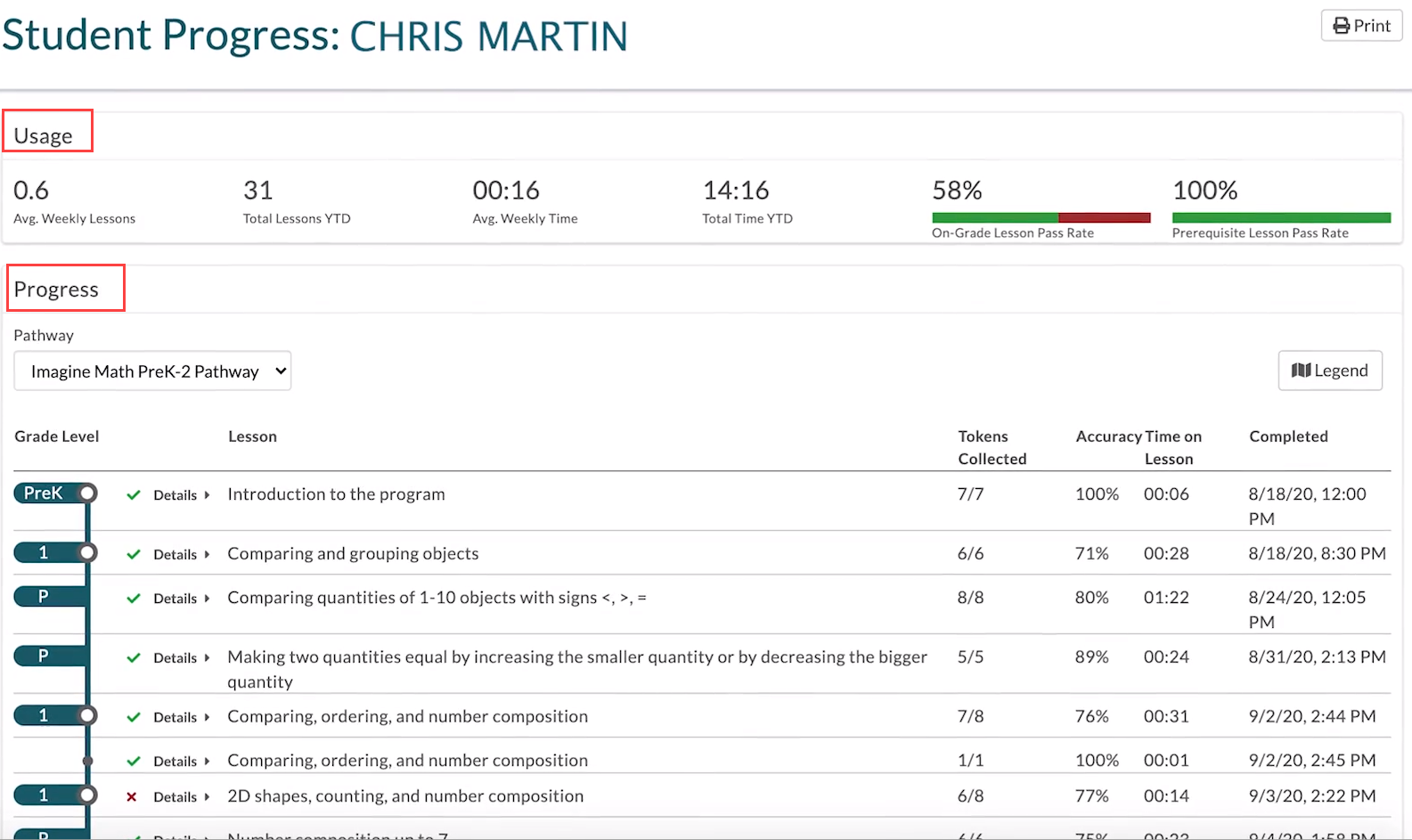### Student Usage

The Usage section provides a summary of how much time a student has spent using Imagine Math PreK-2, as well as their On-Grade Lesson and Prerequisite Lesson pass rates.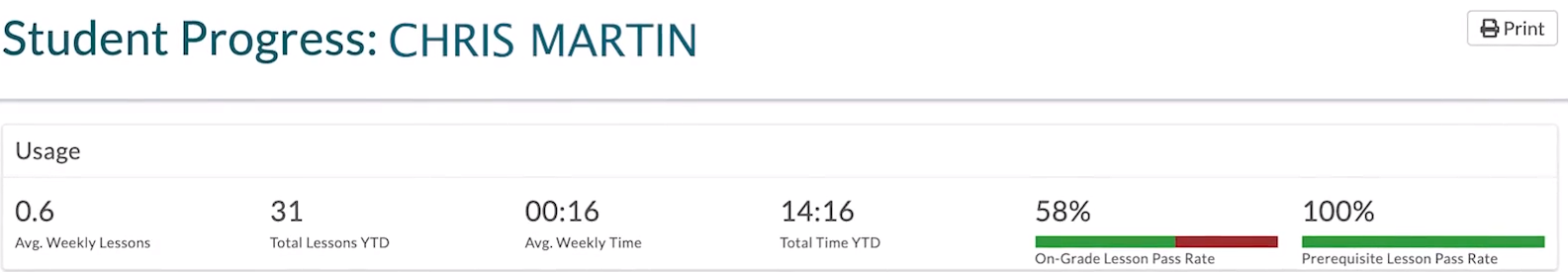Field Description
Avg. Weekly Lessons Shows the average number of lessons the student has completed each week since the beginning of the school year.
Total Lessons YTD Shows the total number of lessons the student has completed year-to-date. Imagine Math recommends students pass 30 lessons per school year.
Avg. Weekly Time Shows the average time the student has spent working on Imagine Math PreK-2 each week.
Total Time YTD Shows the total time the student has spent using Imagine Math PreK-2 year-to-date.
On-Grade Lesson Pass Rate Shows the student's pass rate for on-grade-level lessons for their rostered grade level.
Prerequisite Lesson Pass Rate Shows the student's prerequisite lesson pass rate. Prerequisite lessons support the on-grade-level lessons in a student’s assigned pathway. If Imagine Math determines that a student has not mastered a skill that is covered in a lesson, the student receives a prerequisite lesson to help ensure that they succeed when they receive the on-grade-level lesson.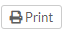Click Print to open a PDF copy of the Student Progress report that can be printed or saved.

### Student Progress

The Progress section provides a map showing each student's progress through the lessons in their individualized pathway. Students are assigned to their pathway after they complete the Benchmark 1 Test.

To view student progress:

1. Choose the pathway that you want to view in the Pathway drop-down list.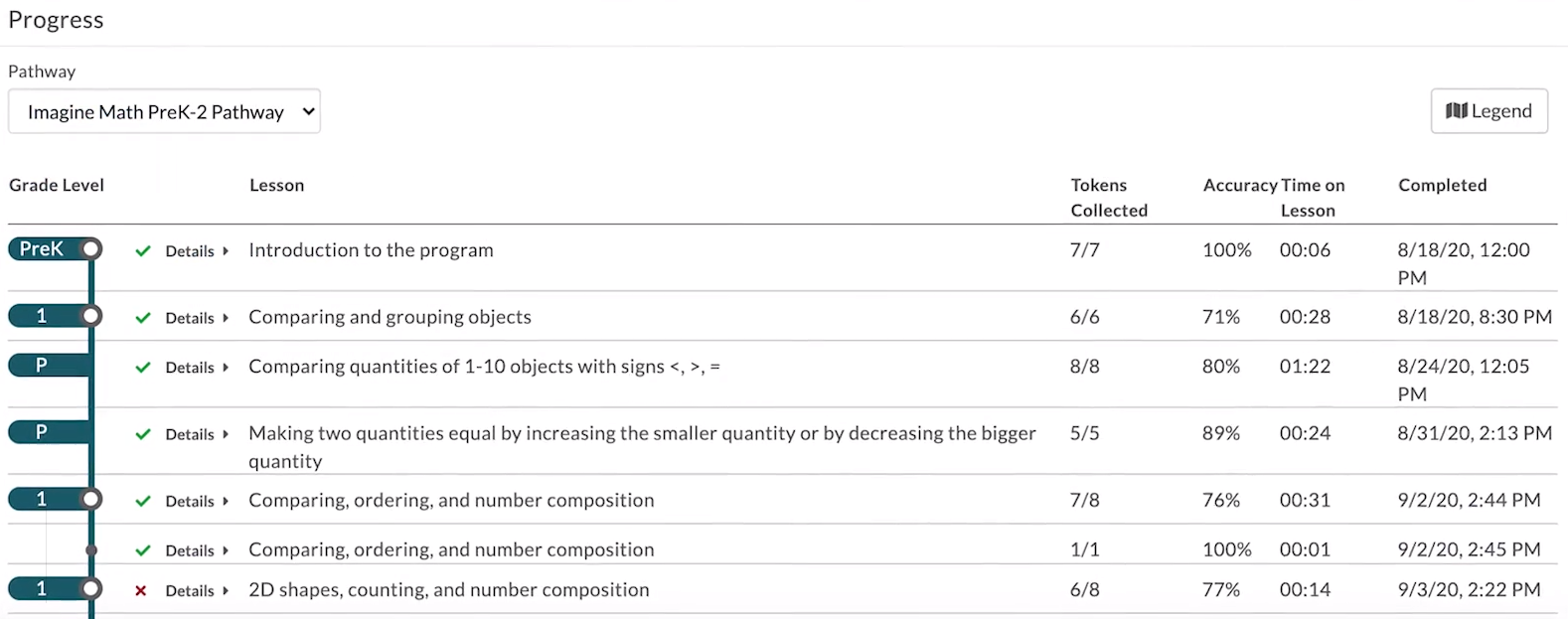Column Description
Grade Level The grade level (Pre-K, K, 1, 2) of the lesson that the student has completed. Based on the student's test scores or pathway, they may receive a prerequisite lesson if they need targeted instruction before a lesson. Prerequisite lessons are indicated by a P in the Grade Level column.
Lesson The lesson's title, which describes the skills taught in the lesson.
Tokens Collected

A fraction displaying the number of tokens the student collected compared to the number of tokens available in the lesson the student completed. The number available is always one greater than the number of activities in the lesson; this is because students earn an additional token for completing the lesson. For example, if there are six activities in a lesson, the score in the Tokens Collected column has a denominator of seven.

To earn a token for each activity, students must demonstrate 50% or higher accuracy on the exercises within the activity.

Note

If a student doesn't earn a token in lesson, they can enter Targeted Review and reattempt those activities to earn the tokens.

Accuracy The percentage of questions that the student answered correctly in the lesson.
Time on Lesson The total amount of time that the student has spent working on the lesson  (if the lesson is still in progress) or the total amount of time that the student took to complete the lesson.

Note

When students are idle for more than two minutes, that time is not counted as Time on Lesson.

Completed The date and time that the student completed the lesson.
2. Click Details to see how the student performed on all the exercises in the lesson. Click an individual exercise to preview its content or to use it for targeted instruction.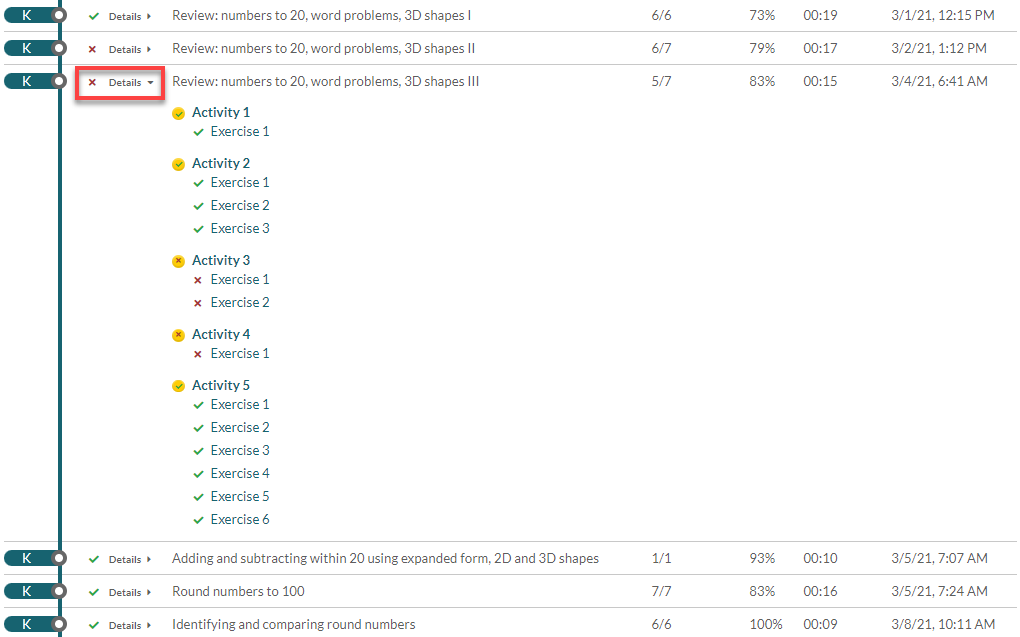Term Description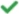Indicates that the student passed the lesson, activity, or exercise. To pass a lesson, a student must earn all tokens in the lesson by scoring at least 50% correct on the exercises in each activity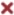Indicates that a student did not pass a lesson, activity, or exercise. Students can retry any lesson or activity that they did not pass by visiting the Treasure Island reward location on the map.

Note

Imagine Math recommends that teachers intervene with students outside of Imagine Math PreK-2 when they do not pass an exercise. After intervention, students can retry the lesson or activity.

3. You can also click Legend for a quick explanation of each icon.